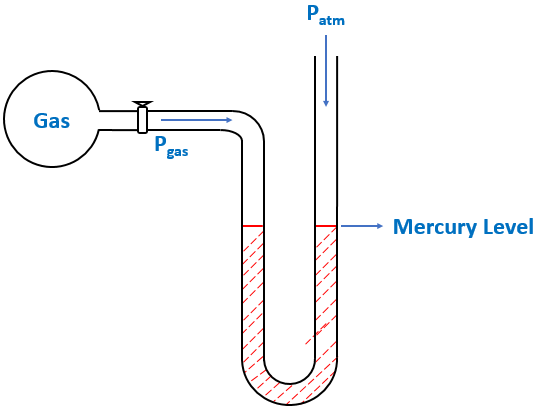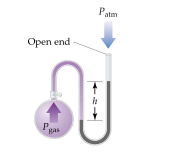# Problem: The atmospheric pressure is 752 mm Hg. What is the pressure (atm) of the enclosed gas when h = 36.0 cm?a) 1.463 atmb) 0.9954 atmc) 1.009 atmd) 1.271 atme) 0.9826 atm

🤓 Based on our data, we think this question is relevant for Professor Bindell's class at UCF.

###### FREE Expert Solution

We’re being asked to determine the pressure of a gas (in atm) using an open-end manometer.

A manometer allows you to determine the pressure of a gas (Pgas) sample by using the atmospheric pressure (Patm) and the difference in the height of mercury (h).

Different conditions for an open-end manometer are illustrated below:

When Pgas is pushing the same force as Patm$\overline{){{\mathbf{P}}}_{\mathbf{g}\mathbf{a}\mathbf{s}}{\mathbf{=}}{{\mathbf{P}}}_{\mathbf{a}\mathbf{t}\mathbf{m}}}$

When Pgas is pushing harder than Patm###### Problem Details

The atmospheric pressure is 752 mm Hg. What is the pressure (atm) of the enclosed gas when h = 36.0 cm?

a) 1.463 atm

b) 0.9954 atm

c) 1.009 atm

d) 1.271 atm

e) 0.9826 atmWhat scientific concept do you need to know in order to solve this problem?

Our tutors have indicated that to solve this problem you will need to apply the Manometer concept. You can view video lessons to learn Manometer. Or if you need more Manometer practice, you can also practice Manometer practice problems.

What is the difficulty of this problem?

Our tutors rated the difficulty ofThe atmospheric pressure is 752 mm Hg. What is the pressure ...as medium difficulty.

How long does this problem take to solve?

Our expert Chemistry tutor, Dasha took 4 minutes and 4 seconds to solve this problem. You can follow their steps in the video explanation above.

What professor is this problem relevant for?

Based on our data, we think this problem is relevant for Professor Bindell's class at UCF.